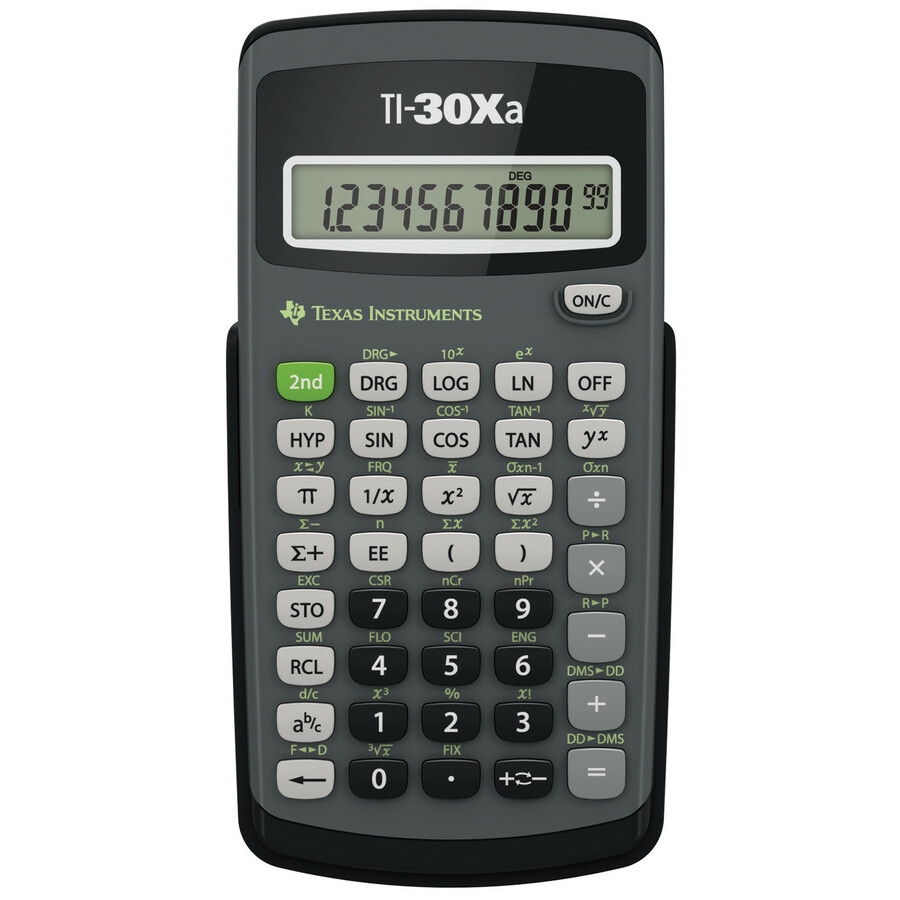Navigate: Home / Hardware / Calculators / Scientific Calculators / Texas Instruments TI-30XA Student Scientific Calculator

# Texas Instruments TI-30XA Student Scientific Calculator

This one-line battery operated model performs basic scientific and trigonometric functions.
*Price: \$19.95
 SKU: TI30XASEVA-X Mfr Part #: Other: TI-30XA School Volume Quote Available?: Yes
Limit one sharing discount per purchase.
 Please Note: This Calculator will ship in plain "White/Brown Box" Packaging"This one-line battery operated model performs basic scientific and trigonometric functions.10-Digit Scientific Calculator is ideal for general math, pre-algebra, algebra 1 and 2, trigonometry and biology. Performs trigonometric functions, logarithms, roots, powers, reciprocals, and factorials. One-variable statistics include results for mean and standard deviation. Calculator also adds, subtracts, multiplies, and divides fractions entered in traditional numerator/denominator format. Conversions include fractions/decimals, degrees/radians/grads, DMS/decimal/degrees, and polar/rectangular. Battery-powered calculator includes slide case.Operations/Functionality: Fraction/decimal conversions Change improper fractions to mixed numbers Automatic simplification of fractions Negation key One constant Combinations and permutations Trigonometry Hyperbolics Logs and antilogs Convert angles from degrees to radians to grads %, x²,x3, ¹/x, x?y, ?, x! Fixed decimal capability One-variable statistics AOS (Algebraic Operating System) Three memory variables Scientific and engineering notation Display & Graphics Number of Display Lines: 1 Number of Display Digits: 10